# RD Sharma Class 9 Solutions Chapter 4 Algebraic Identities VSAQS

## RD Sharma Class 9 Solutions Chapter 4 Algebraic Identities VSAQS

These Solutions are part of RD Sharma Class 9 Solutions. Here we have given RD Sharma Class 9 Solutions Chapter 4 Algebraic Identities VSAQS

Question 1.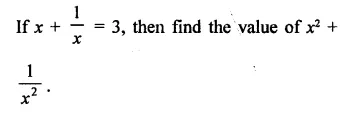Solution: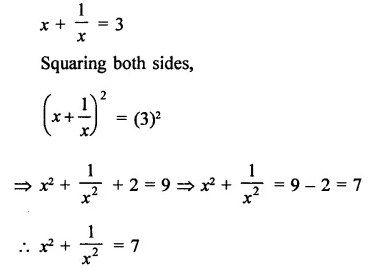Question 2.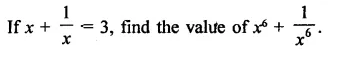Solution: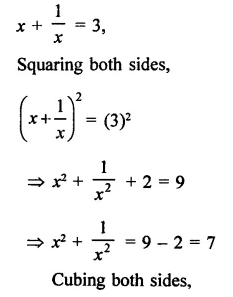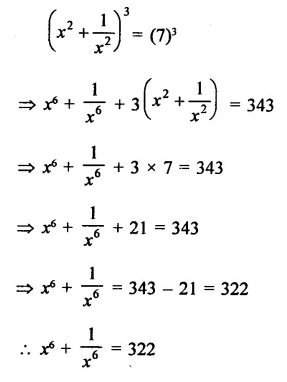Question 3.
If a + b = 7 and ab = 12, find the value of a2 + b2.
Solution:
a + b = 7, ab = 12
Squaring both sides,
(a + b)2 = (7)2
⇒  a2 + b2 + 2ab = 49
⇒  a2 + b2 + 2 x 12 = 49
⇒ a2 + b2 + 24 = 49
⇒ a2 + b2 = 49 – 24 = 25
∴ a2 + b2 = 25

Question 4.
If a – b = 5 and ab = 12, find the value of a2 + b2 .
Solution:
a – b = 5, ab = 12
Squaring both sides,
⇒ (a – b)2 = (5)2
⇒  a2 + b2 – 2ab = 25
⇒  a2 + b2 – 2 x 12 = 25
⇒  a2 + b2 – 24 = 25
⇒  a2 + b2 = 25 + 24 = 49
∴ a2 + b2 = 49

Question 5.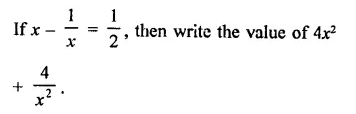Solution: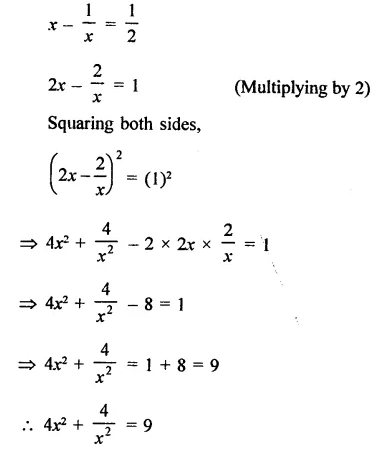Question 6.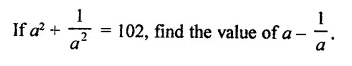Solution: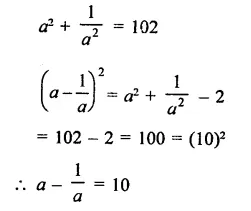Question 7.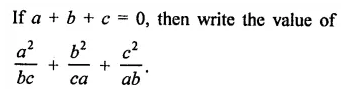Solution: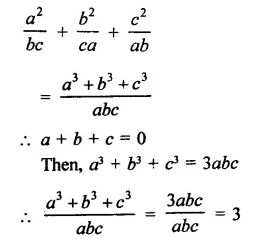Hope given RD Sharma Class 9 Solutions Chapter 4 Algebraic Identities VSAQS are helpful to complete your math homework.

If you have any doubts, please comment below. Learn Insta try to provide online math tutoring for you.